Categories: Quantum information.

Toffoli gate

The Toffoli gate or controlled-controlled-NOT (CCNOT) gate is a logic gate that is reversible (no information is lost) and universal (all reversible logic circuits can be built using Toffoli gates).

It takes three input bits $$A$$, $$B$$ and $$C$$, of which it returns $$A$$ and $$B$$ unchanged, and flips $$C$$ if both $$A$$ and $$B$$ are true. In circuit diagrams, its representation is: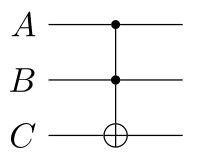This gate is reversible, because $$A$$ and $$B$$ are preserved, and are all you need to reconstruct to $$C$$. Moreover, this gate is universal, because we can make a NAND gate from it: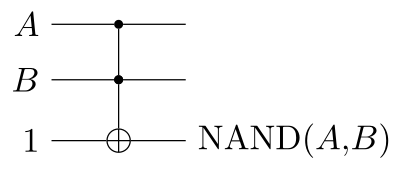A NAND is enough to implement every conceivable circuit. That said, we can efficiently implement NOT, AND, and XOR using a single Toffoli gate too. Note that NOT is a special case of NAND: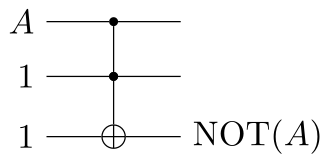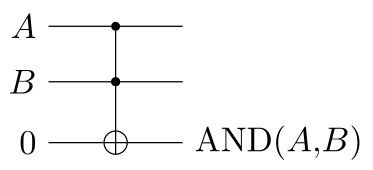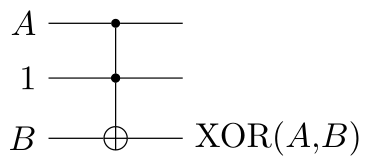Using these, we can, as an example, make an OR gate from three Toffoli gates, thanks to the fact that $$A \lor B = \neg (\neg A \land \neg B)$$, i.e. OR is NAND of NOT $$A$$ and NOT $$B$$: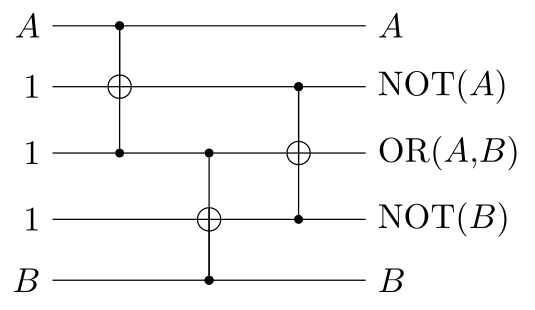Thanks to its reversibility and universality, the Toffoli gate is interesting for quantum computing. Its quantum gate form is often called CCNOT. In the basis $$\ket{A} \ket{B} \ket{C}$$, its matrix is:

\begin{aligned} \boxed{ \mathrm{CCNOT} = \begin{bmatrix} 1 & 0 & 0 & 0 & 0 & 0 & 0 & 0 \\ 0 & 1 & 0 & 0 & 0 & 0 & 0 & 0 \\ 0 & 0 & 1 & 0 & 0 & 0 & 0 & 0 \\ 0 & 0 & 0 & 1 & 0 & 0 & 0 & 0 \\ 0 & 0 & 0 & 0 & 1 & 0 & 0 & 0 \\ 0 & 0 & 0 & 0 & 0 & 1 & 0 & 0 \\ 0 & 0 & 0 & 0 & 0 & 0 & 0 & 1 \\ 0 & 0 & 0 & 0 & 0 & 0 & 1 & 0 \end{bmatrix} } \end{aligned}

If we apply this gate to an arbitrary three-qubit state $$\ket{\psi}$$, it swaps the last two coefficients:

\begin{aligned} \mathrm{CCNOT} \ket{\psi} &= \mathrm{CCNOT} \big( c_{000} \ket{000} + c_{001} \ket{001} + c_{010} \ket{010} + c_{011} \ket{011} \\ &\qquad\qquad\quad\:\; c_{100} \ket{100} + c_{101} \ket{101} + c_{110} \ket{110} + c_{111} \ket{111} \big) \\ &= c_{000} \ket{000} + c_{001} \ket{001} + c_{010} \ket{010} + c_{011} \ket{011} \\ &\quad\,\, c_{100} \ket{100} + c_{101} \ket{101} + c_{111} \ket{110} + c_{110} \ket{111} \end{aligned}

1. J.S. Neergaard-Nielsen, Quantum information: lectures notes, 2021, unpublished.

© Marcus R.A. Newman, a.k.a. "Prefetch". Available under CC BY-SA 4.0.